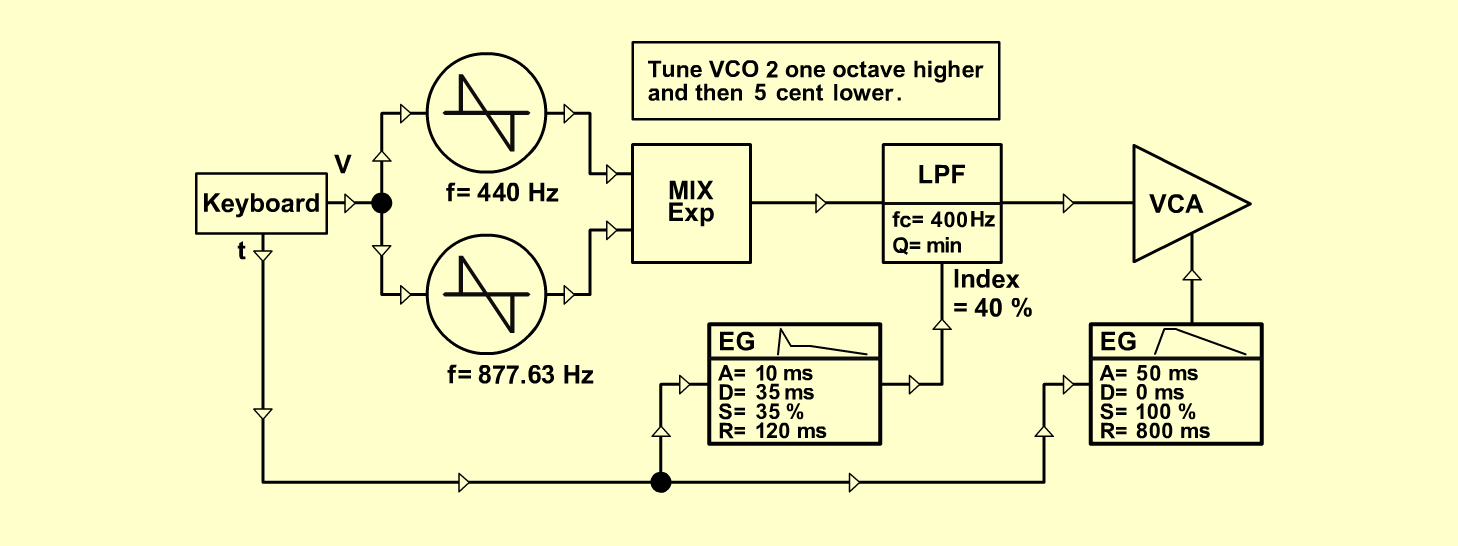StringsInstrumentsD o e p f e r A - 100 Connections: Settings: A-110/1 (Saw)  <=>  A-138 (Input 1) A-110/2 (Saw)  <=>  A-138 (Input 2) A-138exp (Output)  <=>  A-120 (Audio In) A-120 (Audio Out)  <=>  A-131 (Audio In) A-140/1 (Output)  <=>  A-120 (CV 2) A-140/2 (Output)  <=>  A-131 (CV 1) Gate: KB, Seq  <=> A-140/1-2 (Gate) Pitch: KB, Seq  <=>  A-110/1-2 (CV 1) A-138exp (In 1 = 10, In 2 = 10, Out = 10) A-120 (Freq = 4, CV 2 = 4, Lev = 10, Res = 0) A-140/1 (A = 1, D = 1, S = 5.5, R = 4, Range = M) A-140/2 (A = 2.5, D = 1.5, S = 10, R = 7, Range = M) A-131 (Gain = 0, Audio In 1 = 10,  Audio Out = 10) Notes: Tune VCO 2 one octave higher and then 5 cents lower.  For example: VCO 1 = 440 Hz, VCO 2 = 877,63 Hz. Josef MuellerSound samples Pachelbel: Canon in D     2. & 3. inst.: Harpsichord / Moon-Walker Strings